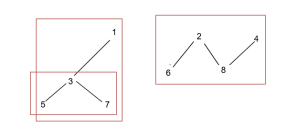# Count number of trees in a forest in C++

Given vertices of a forest ( collection of trees). The goal is to find the number of trees in that forest. We will do this by running a DFS (depth first search) algorithm on the forest.

For Example

## Input

edges = { { 1,3 }, {2,8}, {2,6}, {3,5}, {3,7}, {4,8} }

## Output

Count of number of trees in a forest are: 3

## Explanation

The number of trees that are present in the forest are −Approach used in the below program is as follows

In this approach we apply Depth First search algorithm on the graph recursively. We will increment count if every connected node is marked visited from one source ( which means a tree is formed ).

• Take an integer vertice as a number of vertices on a graph.

• Take a vector vector<int> vec[vertice] for storing vertices as integers.

• Function insert(vector<int> vec[], int parent, int child) takes vec[] and adds an edge between nodes parent and child in that vector.

• Add edge using vec[parent].push_back(child) and vec[child].push_back(parent).

• Function recurred(int temp, vector<int> vec[], vector<bool> &check) applies DFS on graph from starting vertex temp.

• Array check[temp] is used to set nodes as visited/unvisited using true/false.

• Traverse vector vec[] using for loop and if check[vec[temp][i]] is false then call recurred(vec[temp][i], vec, check) recursively for connected nodes.

• Function Trees_Forest(vector<int> vec[], int vertice) takes vec[] and returns the count of trees in the forest given as adjacency list in vec[].

• Take initial count of forests as 0.

• Take vector<bool> check(vertice, false) to mark vertices of graph as visited.

• Traverse all vertices using for loop.

• If check[i] is false then apply dfs using recurred(i, vec, check) and increment count.

• At the end of all loops return count as result.

## Example

Live Demo

#include<bits/stdc++.h>
using namespace std;
void insert(vector<int> vec[], int parent, int child){
vec[parent].push_back(child);
vec[child].push_back(parent);
}
void recurred(int temp, vector<int> vec[], vector<bool> &check){
check[temp] = true;
int size = vec[temp].size();
for(int i = 0; i < size; i++){
if (check[vec[temp][i]] == false){
recurred(vec[temp][i], vec, check);
}
}
}
int Trees_Forest(vector<int> vec[], int vertice){
int count = 0;
vector<bool> check(vertice, false);
for(int i = 0; i < vertice; i++){
if(check[i] == false){
recurred(i, vec, check);
count++;
}
}
return count;
}
int main(){
int vertice = 9;
vector<int> vec[vertice];
insert(vec, 1, 3);
insert(vec, 2, 8);
insert(vec, 2, 6);
insert(vec, 3, 5);
insert(vec, 3, 7);
insert(vec, 4, 8);
cout<<"Count of number of trees in a forest are: "<<Trees_Forest(vec, vertice);
return 0;
}

## Output

If we run the above code it will generate the following output −

Count of number of trees in a forest are: 3